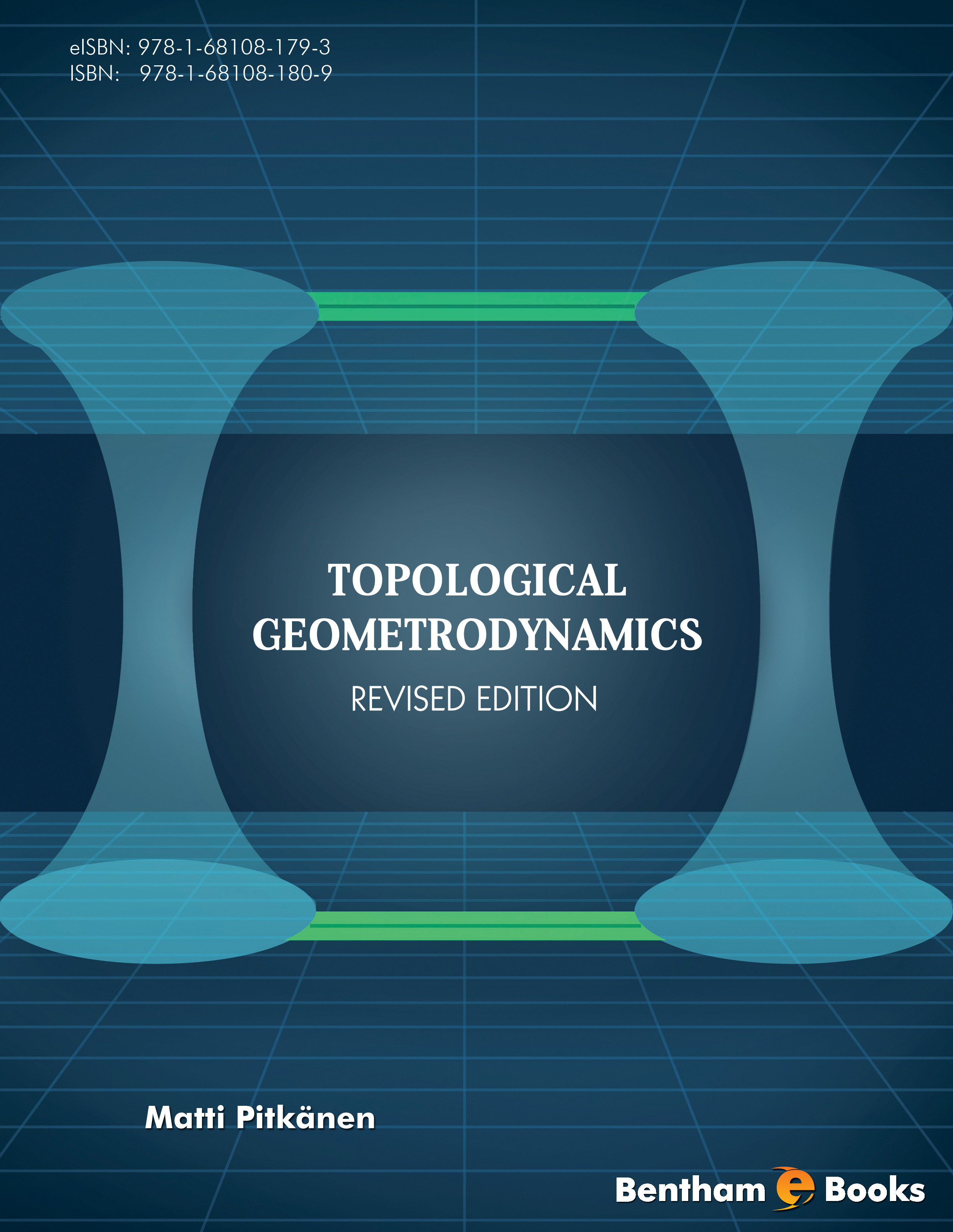### Physocs As Generalized Number Theory

#### Abstract

There are two basic approaches to the construction of quantum TGD. The first approach relies on the vision of quantum physics as infinite-dimensional Kahler geometry for the &quot;world of classical worlds&quot; identified as the space of 3-surfaces in in certain 8-dimensional space. Essentially a generalization of the Einstein&#039;s geometrization of physics program is in question. &lt;/p&gt;&lt;p&gt; The second vision identifies physics as a generalized number theory and involves three threads: various p-adic physics and their fusion together with real number based physics to a larger structure, the attempt to understand basic physics in terms of classical number fields (in particular, identifying associativity condition as the basic dynamical principle), and infinite primes whose construction is formally analogous to a repeated second quantization of an arithmetic quantum field theory. &lt;/p&gt;&lt;p&gt; 1. p-Adic physics and their fusion with real physics &lt;/p&gt;&lt;p&gt; The basic technical problems of the fusion of real physics and various p-adic physics to single coherent whole relate to the notion of definite integral both at space-time level, imbedding space level and the level of WCW (the &quot;world of classical worlds&quot;) . The expressibility of WCW as a union of symmetric spaces leads to a proposal that harmonic analysis of symmetric spaces can be used to define various integrals as sums over Fourier components. This leads to the proposal the p-adic variant of symmetric space is obtained by a algebraic continuation through a common intersection of these spaces, which basically reduces to an algebraic variant of coset space involving algebraic extension of rationals by roots of unity. This brings in the notion of angle measurement resolution coming as = 2 =pn for given p-adic prime p. Also a proposal how one can complete the discrete version of symmetric space to a continuous p-adic versions emerges and means that each point is effectively replaced with the p-adic variant of the symmetric space identifiable as a p-adic counterpart of the real discretization volume so that a fractal p-adic variant of symmetric space results.....

Total Pages: 517-675 (159)